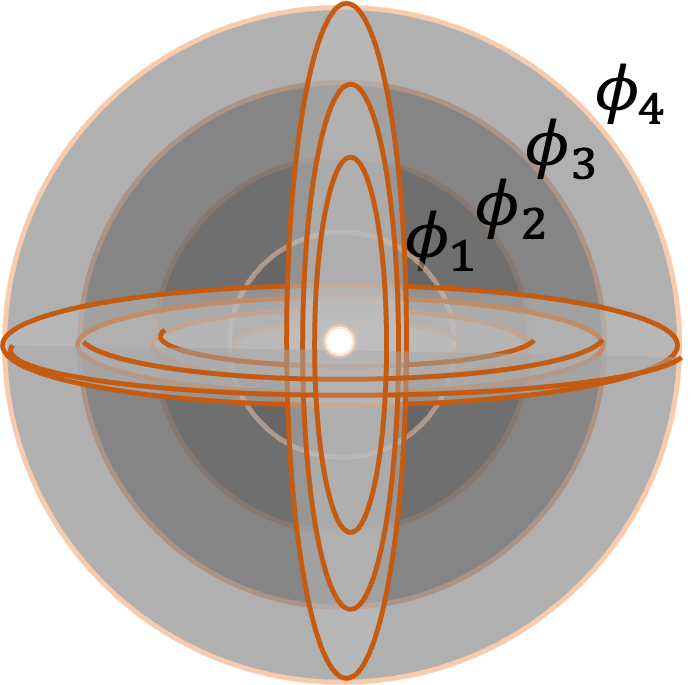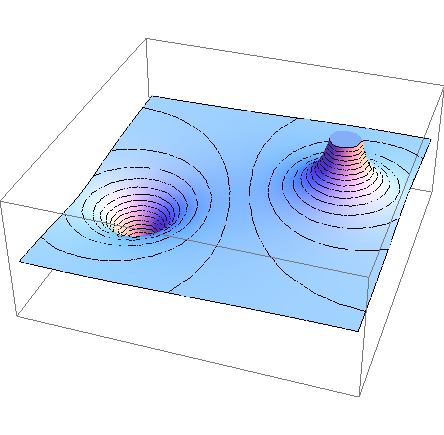## Section31.2Electric Potential Map

We can use Eq. (31.1.4), reproduced here, to map out a space with value of electric potential at representative points.

\begin{equation*} \phi_P = \dfrac{ W_{\text{ref}\rightarrow P}^{\text{by you}} }{Q} = \dfrac{ U_P - U_\text{ref} }{Q}. \end{equation*}

Basically, we choose a test charge $Q$ and measure work required by a force that balances electric force on $Q$ when we bring the test charge from a reference point to the point under study. We then divide the measured work by the amount of the test charge. We do this for all representative points in space to build the map.

The electric potential maps contain surfaces of same potential values - they are called equipotential surfaces. It is a common practice to organize electric potential maps by presenting equipotential surfaces. This is akin to the contour map of a terrain on Earth - that one, actually gives equipotential lines of graviational potential.

Electric potential map of a point particle

Let's work out the potential map of a positive point charge $+ q\text{.}$ Since the force on a test charge $Q$ by the electric field of this charge is spherically symmetric, we expect the potential values to depend only on the distance from the charge as shown in Figure 31.2.1. This gives us equipotential surfaces to be spherical in shape. The potential at the charge is infinite and drops off as $1/\text{distance}$ from the source.Figure 31.2.1. Potential map of a point charge. Equipotential points fall on spherical surfaces around the charge. Each equipotential surface is labeled with the value of the electric potential. A cross-section of the map will show equipotential contour lines that are circles here.

Electric potential map of two oppositely charged point charges

The equipotential map of a system of two oppositely charged point particles, $+q$ and $-q\text{,}$ is more complicated. Figure 31.2.2 shows a three-dimensional surface plot of their joint potential. The potential at positive charge is $+\infty$ and at the negative charge is $-\infty$ with zero far away from the charges. Equipotential lines shown in the cross-section in the figure are curved lines. Near the charges, they are circular. At the plane that is equidistant from the two charges, potential is zero since positive potential from the positive charge and negative potential from the negative charge cancel out there.Figure 31.2.2. Potential map of two opositely charged point charges. The dip is at the negative charge and the peak at the psitive charge. If you take a horizontal plane that contains the two charges, we will obtain ovals around each charge as shown by lines in the figure.